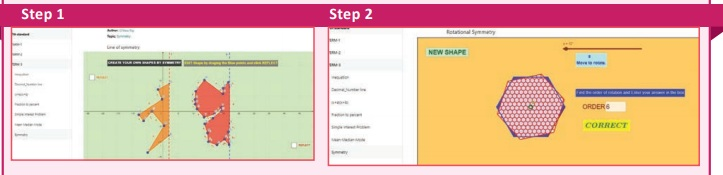Home | | Maths 7th Std | Summary

# Summary

7th Maths : Term 3 Unit 4 : Geometry : Summary

Summary

● A transformation is a specific set of rules that change the preimage onto the image.

● A translation is a transformation that moves all points of a figure in the same distance in the same direction.

● In horizontal, the right-side movement is denoted by → and the left side movement is denoted by ←.

● In vertical, the upside movement is denoted byand the downward movement is denoted by .

● A reflection is a transformation that “flips” or “reflects” a figure about a line.

● A rotation is a transformation that turns every point of the pre-image through a specified angle and direction about a point. The fixed point is called the centre of rotation. The angle is called the angle of rotation.

● A rotation is also called a turn.

● The default direction of a rotation is the anti-clockwise direction.

● Rotation of 360° is called a full turn, rotation of 180° is called a half turn, rotation of 90° is called a quarter turn.

● The collection of all the points in a plane, which are at a fixed distance from a fixed point in the plane, is called a circle. The fixed point is called the centre of the circle and the fixed distance is called the radius of the circle.

● If two points on a circle are joined by a line segment, then the line segment is called a chord of the circle.

● The chord, which passes through the centre of the circle, is called a diameter of the circle.

● Circles drawn in a plane with a common centre and different radii are called concentric circles.

● The area between the two concentric circles is known as circular ring

● Width of the circular ring (w) = r2r1

ICT Corner

Geometry

Expected outcomeStep – 1

Open the Browser and type the URL Link given below (or) Scan the QR Code. GeoGebra work sheet named “Symmetry” will open. Drag the points on left side of the line to change the shape. Click “REFLECT” check box to see the symmetricity.

Step – 2

Scroll down to see another worksheet called “Rotational symmetry”. Move the slider to rotate the shape and find the order of rotation and enter in the box given. By pressing enter, you can check whether your answer is correct. Click on “NEW SHAPE” and repeat the process.